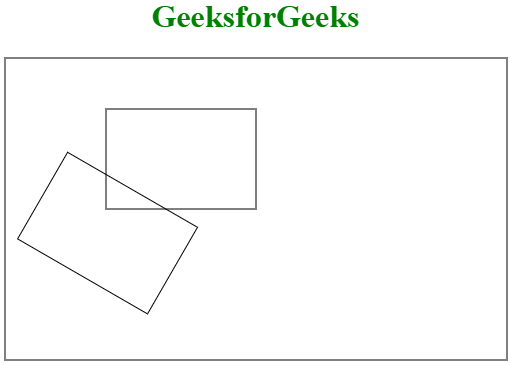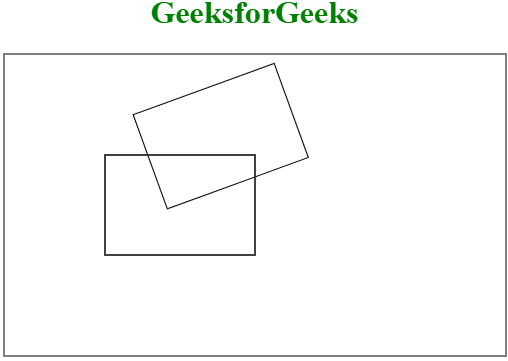# HTML canvas rotate() Method

The HTML canvas rotate() Method is used to rotate the drawing by a given angle. Note that the rotation will only work on the canvas that was made after the rotation was done.

Syntax:

`context.rotate(angle)`

Parameter Values:

• angle: It stores the rotation angle in radians. If the angle is given in the form of a degree then it converts into a radian by using the formula degree*Math.PI/180.

Example 1:

## html

 ` ` `<``html``> `   `<``head``> ` `    ``<``title``> ` `        ``HTML canvas rotate() Method` `    `` ` ` `   `<``body``> ` `<``center``>` `<``h1` `style="color:green">GeeksforGeeks` `    ``<``canvas` `id="GFG" width="500"` `            ``height="300" style="border:2px solid gray;"> ` ` `   `    ``<``script``> ` `        ``var geeks = document.getElementById("GFG"); ` `        ``var context = geeks.getContext("2d"); ` `        ``context.rect(100, 100, 150, 100);//actual rectangle` `        ``context.stroke(); ` `        `  `        ``context.rotate((-20) * Math.PI / 180);` `        ``context.rect(100, 100, 150, 100);//rotate rectangle` `        ``context.stroke();` `    `` ` `` ` `   ``

Output:Example 2:

## html

 ` ` `<``html``> `   `<``head``> ` `    ``<``title``> ` `        ``HTML canvas rotate() Method` `    `` ` ` `   `<``body``> ` `<``center``>` `<``h1` `style="color:green">GeeksforGeeks` `    ``<``canvas` `id="GFG" width="500"` `            ``height="300" style="border:2px solid gray;"> ` ` `   `    ``<``script``> ` `        ``var geeks = document.getElementById("GFG"); ` `        ``var context = geeks.getContext("2d"); ` `        ``context.rect(100, 100, 150, 100);//actual rectangle` `        ``context.stroke(); ` `        `  `        ``context.rotate((-20) * Math.PI / 180);` `        ``context.rect(100, 100, 150, 100);//rotate rectangle` `        ``context.stroke();` `    `` ` `` ` `   `                                      `

Output:Supported Browsers: